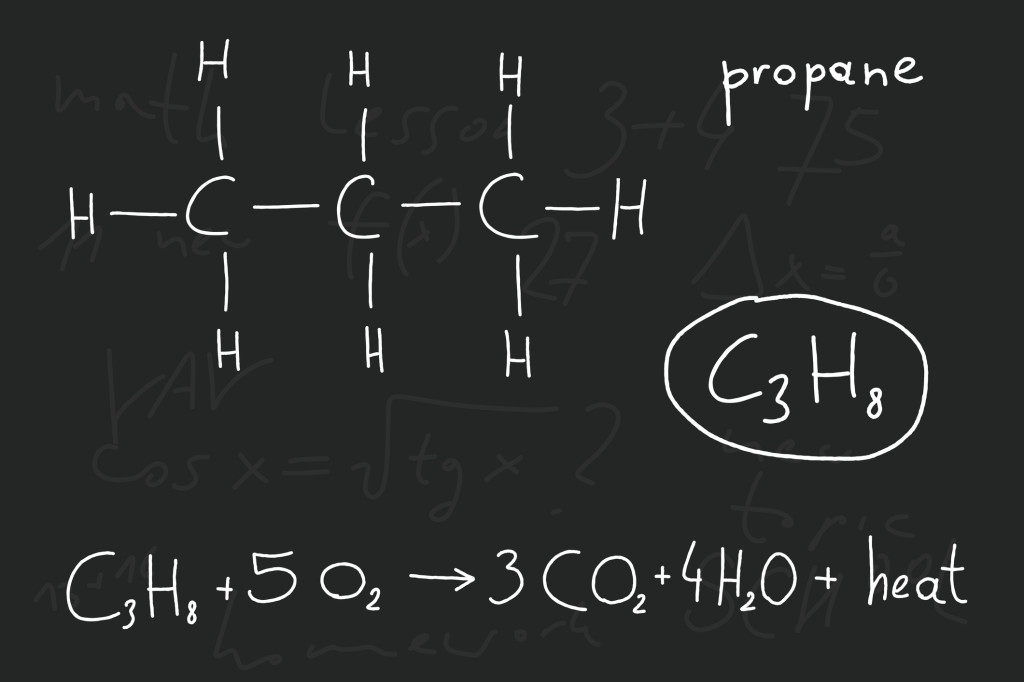# Balancing Chemical Equations

When you want to do chemical experiments, or to produce chemicals in certain amounts, you need to know both how to make them and how much of their “ingredients” you will require. In chemistry, this is called stoichiometry, or the measure of the elements or molecules that are involved in a chemical reaction. Find out how to balance chemical equations!

One important rule in chemistry is that matter isn’t created or destroyed in chemical reactions, only changed in form. And this means that chemical reactions, and the formulas that describe them, must be balanced.

Let’s consider a simple reaction between methane and oxygen that would happen in the burning of natural gas to power and engine. We would take propane (C3H8) and oxygen gas (O2), and when they react we come out with carbon dioxide gas (CO2) and water (H2O). The equation would look something like this:
C3H8 + O2 → CO2 + H2O

***BUT you can see that it is not balanced! We started with 3 carbon atoms, 8 hydrogen atoms, and 2 oxygen atoms, however we ended up with 1 carbon, only 3 oxygen, and only 2 hydrogen atoms.

This can’t be right – where did the 2 extra carbon and the other 6 hydrogen atoms go, and how did we get more oxygen?

What happened is that we thought we could take only one molecule each of oxygen gas and propane and react them together. However, the products of the reaction can’t form properly, so we must have the numbers wrong. Can you see how to balance the equation?C3H8 + 5O2                         →        3CO2 + 4H2O    is a balanced equation.

3 carbons                                             3 carbons

8 hydrogens                                       4 H2 (=4 x 2) = 8 hydrogens

5 O2 (= 5 x 2) = 10 oxygens           3 O2 + 4 O (=3 x 2 + 4) = 10 oxygens

You can see that the subtext number in a molecule shows how many atoms of that sort are in one molecule, so in every O2 molecule there are 2 oxygen atoms. If you place a number in front of the molecule, it gets multiplied by every type of atom in that molecule. For example, 2H2O means there are 2 x H 2 = 4 hydrogen atoms and 2 x O = 2 oxygen atoms in 2 water molecules.

Here are some other examples of balanced chemical equations:

CaCO3 → CaO + CO2

2C7H6O2 + 15O2 → 14CO2 + 6H2O

2Al + 2NaOH + 6H2O → 2NaAl(OH)4 + 3H2